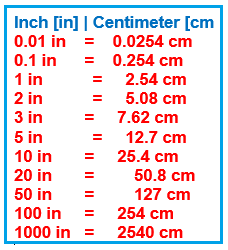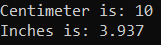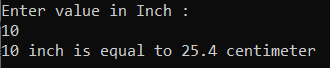# Inches to Centimeters in C#

## Inches to Centimeters in C# with Examples

In this article, I am going to discuss how to Convert Inches to Centimeters in C# with Examples. Please read our previous article, where we discussed the Profit and Loss Program in C#.

##### Inches to Centimeters in C#
1. Inches: An inch is defined as the measurement unit used in Imperial and the US Customary measurement systems. It is the unit of length.
2. Centimeters: The unit centimeter is also used to measure the length, and used in the International System of Units, which is the current form of the metric system.
###### Inches to Centimeters Calculation

1 Inch = 2.54 Centimeters.
1 Centimeter = 0.393701 inches.

Example:
convert 15 inches to cm:15 in = 15 × 2.54 cm = 38.1 cm.

##### Inch to Centimeter Conversion Table##### Logic to Convert Inches to Centimeter in C#

To find the Inches to Centimeter is, simply we need to follow the below steps:-
1)First we will define inches and centimeters.
2)Then we will assign the value of inches.
3)then we will calculate centimeters by using a formula.
The formula of inches to centimeters is the value of 1 inch is approximately equal to 2.54 centimeters. It means that 1 inch = 2.54 cm. To convert inches to the centimeter values, multiply the given inch value by 2.54 cm. Similarly, the conversion of centimeters to inches can proceed as follows
We know that 1 inch to equivalent to 2.54 cm
Therefore, 1 cm = 1 / 2.54
1 cm = 0.393701 inches.
4) And the resulting output will store in the centimeter.

##### Example: Inches to Centimeter Program in C#

The following C# Program will convert centimeters to inches.

```using System;
class Inchestocentimeters
{
public static void Main()
{
double centimeter = 10;
Console.WriteLine("Centimeter is: " + centimeter);
double inch = 0.3937 * centimeter;
Console.WriteLine("Inches is: " + inch);
}
}
```
###### Output:##### Example: Taking input from the user

In the following C# program, we will take the Inches from the user and then calculate the centimeters and print it on the console.

```using System;
public class Inchestocentimeter
{
public static float convert(float a)
{
float cm1;
cm1 = (float)(a * 2.54);
return cm1;
}

public static void Main(string[] args)
{
float inch, cm;
Console.WriteLine("Enter value in Inch : ");